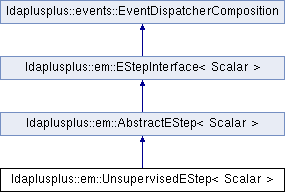LDA++
ldaplusplus::em::UnsupervisedEStep< Scalar > Class Template Reference

#include <UnsupervisedEStep.hpp>

Inheritance diagram for ldaplusplus::em::UnsupervisedEStep< Scalar >:## Public Member Functions

UnsupervisedEStep (size_t e_step_iterations=10, Scalar e_step_tolerance=1e-2, Scalar compute_likelihood=1.0, int random_state=0)

virtual std::shared_ptr< parameters::Parametersdoc_e_step (const std::shared_ptr< corpus::Document > doc, const std::shared_ptr< parameters::Parameters > parameters) overridePublic Member Functions inherited from ldaplusplus::em::AbstractEStep< Scalar >
AbstractEStep (int random_state)

virtual void e_step () overridePublic Member Functions inherited from ldaplusplus::events::EventDispatcherComposition
std::shared_ptr< EventDispatcherInterfaceget_event_dispatcher ()

void set_event_dispatcher (std::shared_ptr< EventDispatcherInterface > dispatcher)Protected Member Functions inherited from ldaplusplus::em::AbstractEStep< Scalar >
bool converged (const Eigen::Matrix< Scalar, Eigen::Dynamic, 1 > &gamma_old, const Eigen::Matrix< Scalar, Eigen::Dynamic, 1 > &gamma, Scalar tolerance)

PRNGget_prng ()

## Detailed Description

### template<typename Scalar> class ldaplusplus::em::UnsupervisedEStep< Scalar >

UnsupervisedEStep implements the classic LDA expectation step.

For each document passed in UnsupervisedEStep::doc_e_step a factorized variational distribution is computed with Dirichlet parameter $$\gamma$$ and multinomial parameters $$\phi$$. The distribution is computed in such a way so that a lower bound of the probability of generating the document given the model parameters (the topics that is) is maximized.

See UnsupervisedEStep::doc_e_step for the mathematics.

 Blei, David M., Andrew Y. Ng, and Michael I. Jordan. "Latent dirichlet allocation." Journal of machine Learning research 3.Jan (2003): 993-1022.

## Constructor & Destructor Documentation

template<typename Scalar >
 ldaplusplus::em::UnsupervisedEStep< Scalar >::UnsupervisedEStep ( size_t e_step_iterations = 10, Scalar e_step_tolerance = 1e-2, Scalar compute_likelihood = 1.0, int random_state = 0 )
Parameters
 e_step_iterations The max number of times to alternate between maximizing for $$\gamma$$ and for $$\phi$$. e_step_tolerance The minimum relative change in the variational parameter $$\gamma$$. compute_likelihood The percentage of documents to compute likelihood for (1.0 means compute for every document) random_state An initial seed value for any random numbers needed

## Member Function Documentation

template<typename Scalar >
 std::shared_ptr< parameters::Parameters > ldaplusplus::em::UnsupervisedEStep< Scalar >::doc_e_step ( const std::shared_ptr< corpus::Document > doc, const std::shared_ptr< parameters::Parameters > parameters )
overridevirtual

Maximize the ELBO w.r.t. to $$\phi$$ and $$\gamma$$.

The following steps are the mathematics that are implemented where $$\beta$$ are the topics, $$i$$ is the topic subscript, $$n$$ is the word subscript, $$w_n$$ is n-th word vocabulary index, $$\alpha$$ is the Dirichlet prior and finally $$\Psi(\cdot)$$ is the first derivative of the $$\log \Gamma$$ function.

1. Repeat following steps until convergence
2. $$\phi_{ni} \propto \beta_{iw_n} \exp(\Psi(\gamma_i))$$
3. $$\gamma_i = \alpha_i + \sum_n^N \phi_{ni}$$
Parameters
 doc A single document parameters An instance of class Parameters, which contains all necessary model parameters for e-step's implementation
Returns
The variational parameters for the current model, after e-step is completed

Implements ldaplusplus::em::EStepInterface< Scalar >.

The documentation for this class was generated from the following files: